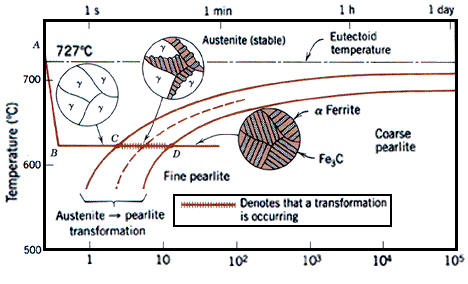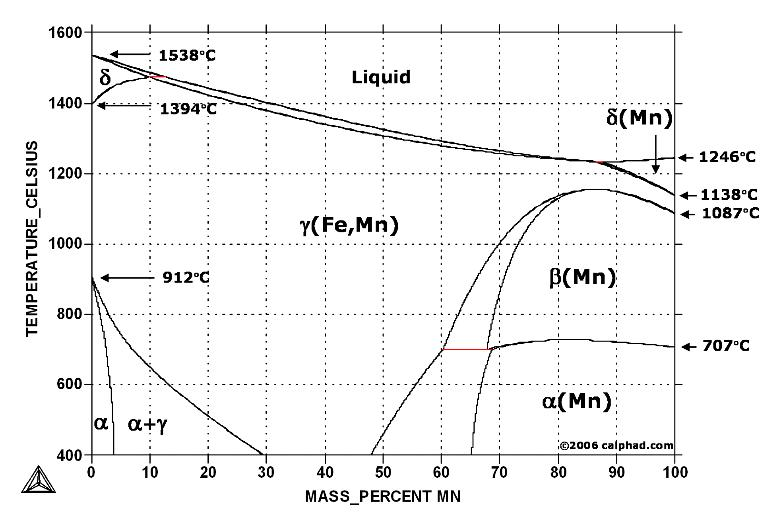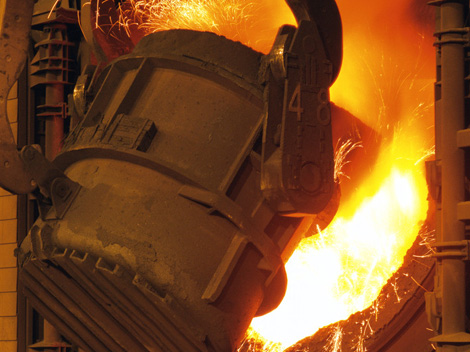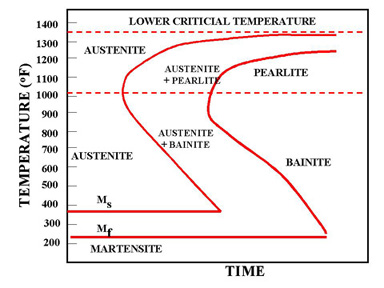Fe-Fe3C Phase Diagram, Materials Science and Metallurgy, 4th ed., Pollack, Prentice-Hall, 1988

Figure above  shows the equilibrium diagram for combinations of carbon in a solid solution of iron. The diagram shows iron and carbons combined to form Fe-Fe3C at the 6.67%C end of the diagram. The left side of the diagram is pure iron combined with carbon, resulting in steel alloys. Three significant regions can be made relative to the steel portion of the diagram. They are the eutectoid E, the hypoeutectoid A, and the hypereutectoid B. The right side of the pure iron line is carbon in combination with various forms of iron called alpha iron (ferrite), gamma iron (austenite), and delta iron. The black dots mark clickable sections of the diagram.

Allotropic changes take place when there is a change in crystal lattice structure. From 2802º-2552ºF the delta iron has a body-centered cubic lattice structure. At 2552ºF, the lattice changes from a body-centered cubic to a face-centered cubic lattice type. At 1400ºF, the curve shows a plateau but this does not signify an allotropic change. It is called the Curie temperature, where the metal changes its magnetic properties.

Two very important phase changes take place at 0.83%C and at 4.3% C. At 0.83%C, the transformation is eutectoid, called pearlite.
gamma (austenite) -> alpha + Fe3C (cementite)
At 4.3% C and 2066ºF, the transformation is eutectic, called ledeburite.
L(liquid) -> gamma (austenite) + Fe3C (cementite)

Problem Solving :

1. Given the Fe-Fe3C phase diagram above, calculate the phases present at the eutectoid composition line at:

a. T = 3000ºF

b. T = 2200ºF

c. T = 1333ºF

d. T = 410ºF

2. Calculate the phases in the cast-iron portion of the diagram at the eutectic composition of 4.3% C in combination with 95.7% ferrite at:

a. T = 3000ºF

b. T = 1670ºF

c. T = 1333ºF

3. A eutectoid steel (about 0.8%C) is heated to 800ºC (1472ºF) and cooled slowly through the eutectoid temperature. Calculate the number of grams of carbide that form per 100g of steel.

4. Determine the amount of pearlite in a 99.5% Fe-0.5%C alloy that is cooled slowly from 870ºC given a basis of 100g of alloy.

Solution :
1. a. T = 3000ºF. Since the composition E is eutectoid, the carbon content is 0.83%.
b. T = 2200ºF. At this temperature, austenite exists as a single-phase solid.
c. T = 1333ºF. Two phases exist, ferrite and austenite.
The percentages are determined by the lever rule: X ÷ (X+Y) = (Cy-C) ÷ (Cy-Cx).
proeutectoid ferrite = (0.83 - 0.18) / (0.83 - 0.025) x 100 = 80.7%
austenite = (0.18 - 0.025) / (0.83 - 0.025) x 100 = 19.3%
d. T = 410ºF. A small amount of cementite will precipitate following the solubility line from 0.025% C at 1333ºF
to 0.008% C at room temperature.
The overall percentages of ferrite   and cementite are:
ferrite = (6.67 - 0.18) / (6.67 - 0.01) x 100 = 97.4
cementite = (0.18 - 0.01) / (6.67 - 0.01) x 100 = 2.6%
2. a. T = 3000ºF. At this temperature, the eutectic is all liquid.
b. T = 1670ºF. Since there is a change of the solubility line, there will be a change in the austenite composition, that            will transform to the eutectoid at 1333ºF. The overall austenite and cementite composition will be:
austenite = (6.67 - 4.3) / (6.67 - 1.2) x 100 = 43.3%
cementite = (4.3 - 1.2) / (6.67 - 1.2) x 100 = 56.7%
c. T = 1333ºF. At this temperature the austenite has a eutectoid composition and will transform into pearlite. The                 compositions of proeutectoid cementite and austenite are:
eutectoid austenite = (6.67 - 4.3) / (6.67 - 0.83) x 100 = 40.6%
proeutectoid cementite = (4.3 - 0.83) / (6.67 - 0.83) x 100 = 59.4%
The austenite has a eutectoid composition that is:
eutectoid ferrite = (6.67 - 0.83) / (6.67 - 0.025) x 100 = 88%
eutectoid cementite = (0.83 - 0.025) / (6.67 - 0.025) x 100 = 12%
3. Interpolate between alpha (0.02%C) and Fe3C (6.7%) at 1333ºF
Carbide = (0.8 - 0.02) / (6.7 - 0.02) x 100 = 12g
4. Since pearlite originates from austenite of eutectoid composition, determine the amount of gamma just prior to the         eutectoid reaction.
From 870ºC - 780ºC : 100g austenite with 0.5% C
From 780ºC - 727ºC : ferrite separates from austenite and the carbon content of the austenite increases to about             0.8% C
At 727ºC (+) : proeutectoid ferrite: composition of ferrite = 0.02% C, amount of carbon = 38g
Gamma that transforms to pearlite: compostion of austenite = 0.8% C. Amount of austenite = 62g.
At 727ºC (-): Amount of pearlite = 62g.

### You might also likeFe-Fe3C T-T-T Diagram   Fe-Fe3C T-T-T Diagram, Adapted from...Phase Diagrams Fe-Mn, Fe-Co, Fe-Mo   In pure iron, the A4 (1394 °C) and...Metallurgy Glossary Metallurgy Glossary Activity: A function...Time-Temperature-Transformation (TTT ) Diagram T (Time) T(Temperature) T(Transformation)...

### Related Post

• Austenite (Gamma Iron)
Austenite, also known as gamma phase iron is a metallic non-magnetic allotrope of iron or a solid solution of iron, with...